# Calculus 2 : Series in Calculus

## Example Questions

← Previous 1 3 4 5 6 7 8 9 32 33

### Example Question #1 : Geometric Series

Consider: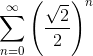.   Will the series converge or diverge? If converges, where does this coverge to?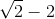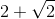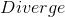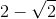Explanation:

This is a geometric series.  Use the following formula, whereis the first term of the series, andis the ratio that must be less than 1.  Ifis greater than 1, the series diverges.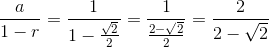Rationalize the denominator.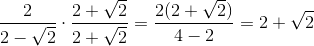### Example Question #2781 : Calculus Ii

Consider the following summation: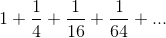.  Does this converge or diverge?  If it converges, where does it approach?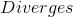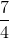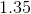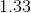Explanation:

The problem can be reconverted using a summation symbol, and it can be seen that this is geometric.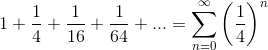Since the ratio is less than 1, this series will converge.  The formula for geometric series is: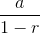whereis the first term, andis the common ratio.  Substitute these values and solve.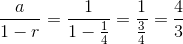### Example Question #1 : Geometric Series

A worm crawls up a wall during the day and slides down slowly during the night. The first day the worm crawls one meter up the wall. The first night the worm slides down a third of a meter. The second day the worm regains one third of the lost progress and slides down one third of that distance regained on the second night. This pattern of motion continues...

Which of the following is a geometric sum representing the distance the worm has travelled after12-hour periods of motion? (Assuming day and night are both 12 hour periods).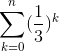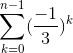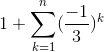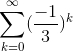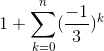Explanation:

The sum must be alternating, and after one period you should have the worm at 1m. After two periods, the worm should be at 2/3m. There is only one sum for which that is true.

### Example Question #1 : Series And Functions

Which of the following is a correct representation of the sum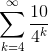in +... notation?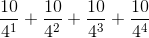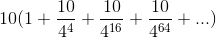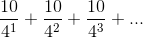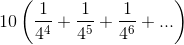Explanation:

By writing out the first few terms and factoring out a 10, we can arrive at a correct representation of the sum in +... notation.

The sum of the first term where k=4 is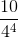.

The term when k=5 is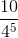.

The term when k=6 is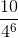.

Creating the summation we get,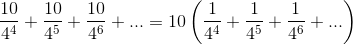### Example Question #1 : Series And Functions

One technique for determining whether a series converges is comparing it to a related improper integral.

Which of the following is the most correct proof of convergence for the series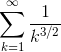?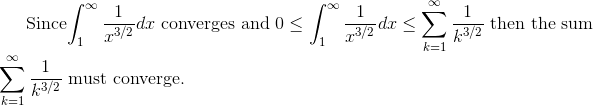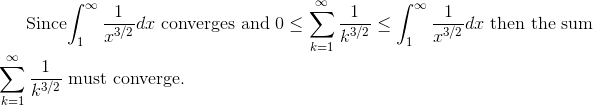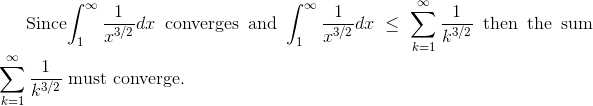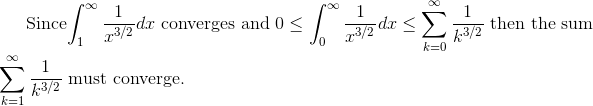Explanation:

In the spirit of the direct comparison test, we can compare the convergent improper integral to the infinite sum, such that the sum is less than the convergent integral by interpreting the sum as a right hand Riemann sum. Note that you can depict the sum as either, with the property that in one case you get information about its convergence or divergence and in the other case you cannot say anything. We want to make sure for correctness that we assert the sum and integral are both positive, as a condition for making the comparison.

By definition of the Comparison Test

Given that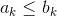where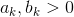.

If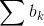converges, then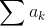also converges.

### Example Question #1 : Series In Calculus

To what value does the sumconverge?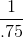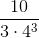Explanation:

The first term of the series is, and the common ratio is, so we can rewrite the series in the form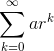and use the formulato arrive at the correct answer.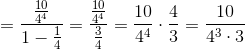### Example Question #4 : Series And Functions

Given that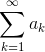converges, what can you say about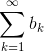where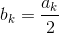and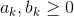.

The sum must converge.

Not enough information.

The sum is zero.

The sum must diverge.

The sum must converge.

Explanation:

The sum must converge by the direct comparison test. All terms are positive and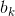is smaller than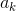for all k. Therefore by definition the sum must converge.

### Example Question #5 : Series And Functions

A worm crawls up a wall during the day and slides down slowly during the night. The first day the worm crawls one meter up the wall. The first night the worm slides down a third of a meter. The second day the worm regains one third of the lost progress and slides down one third of that distance regained on the second night. This pattern of motion continues...

Which of the following is a correct expression for the distance the worm has travelled after the second night?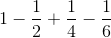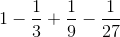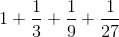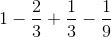Explanation:

This is just a finite sum of the distance the worm travelled and fell each day.

The first day the worm traveled a postive one meter.

Over the night he lost one third of a meter. This can be translated into math terms as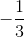.

He continued this ratio progression each day.

### Example Question #6 : Series And Functions

A worm crawls up a wall during the day and slides down slowly during the night. The first day the worm crawls one meter up the wall. The first night the worm slides down a third of a meter. The second day the worm regains one third of the lost progress and slides down one third of that distance regained on the second night. This pattern of motion continues...

How high off the ground will the worm eventually end up if he keeps at it forever?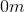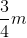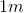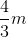Explanation:

This is a geometric series with an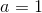and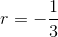so will converge to: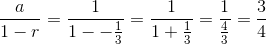### Example Question #1 : Series And Functions

Which is the correct Maclaurin series representation for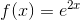?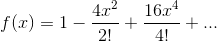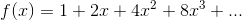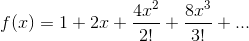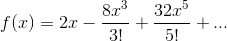Explanation:

The general form for the Maclaurin series for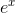is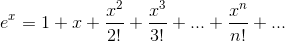To find the series representation for, simply substitutein place ofin the series for.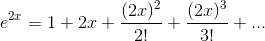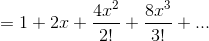← Previous 1 3 4 5 6 7 8 9 32 33

### All Calculus 2 Resources